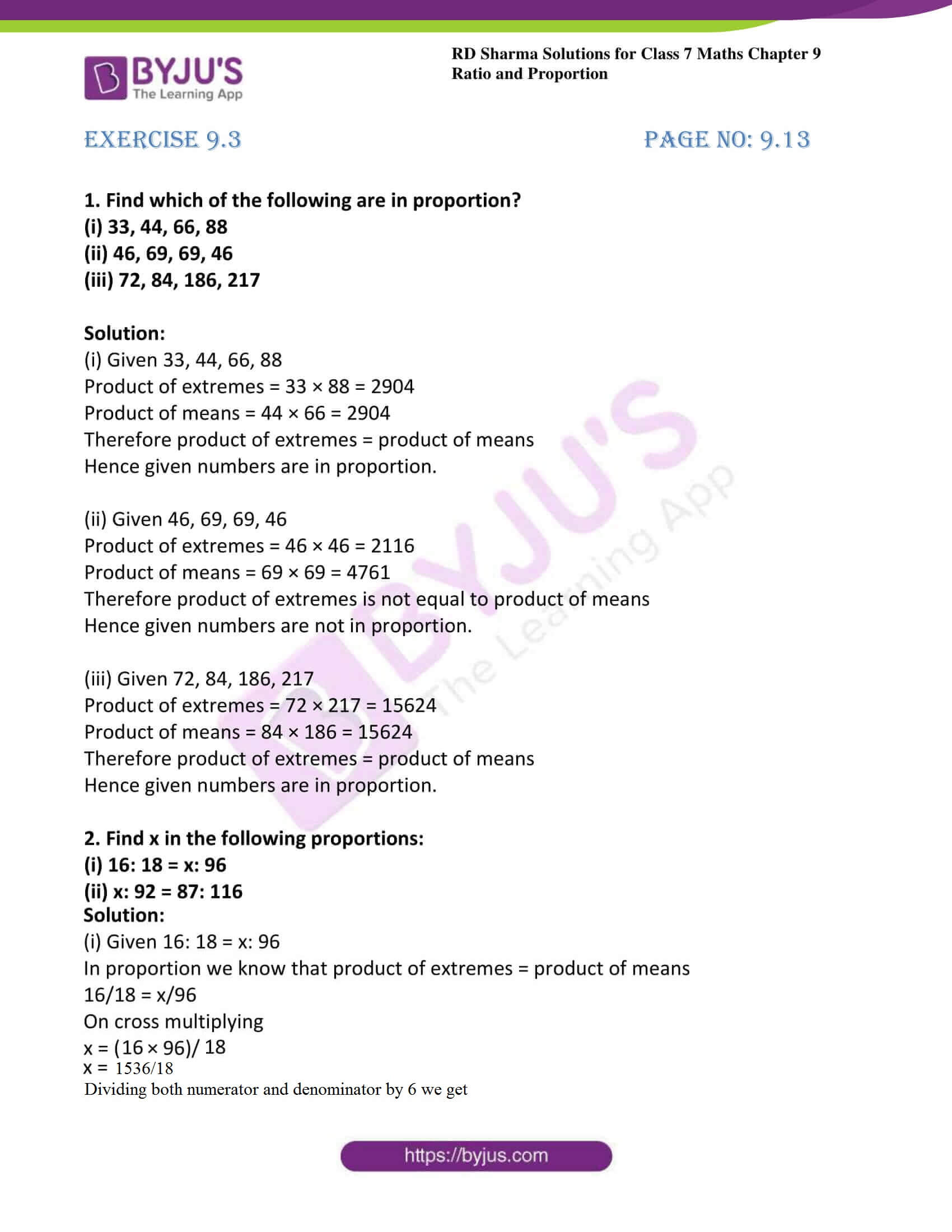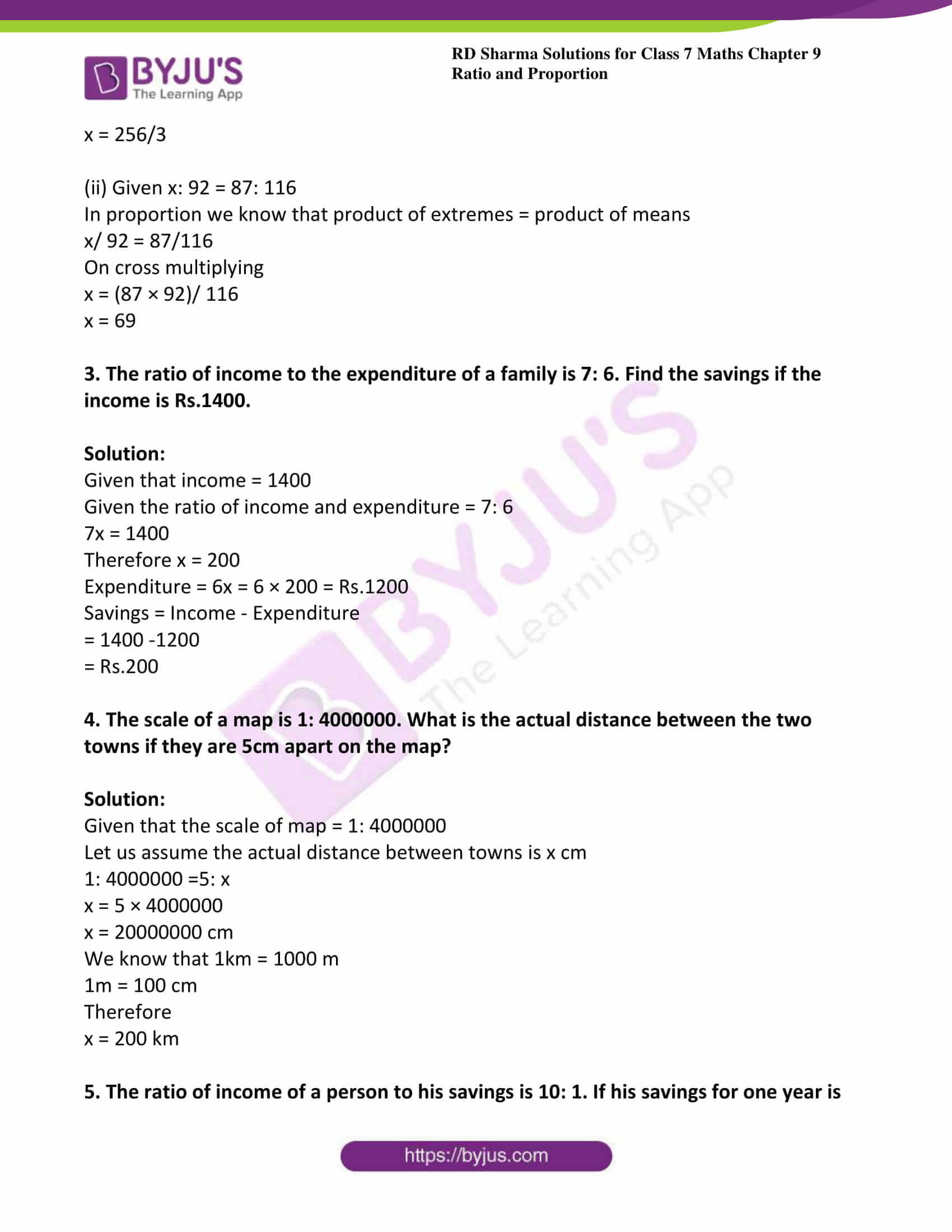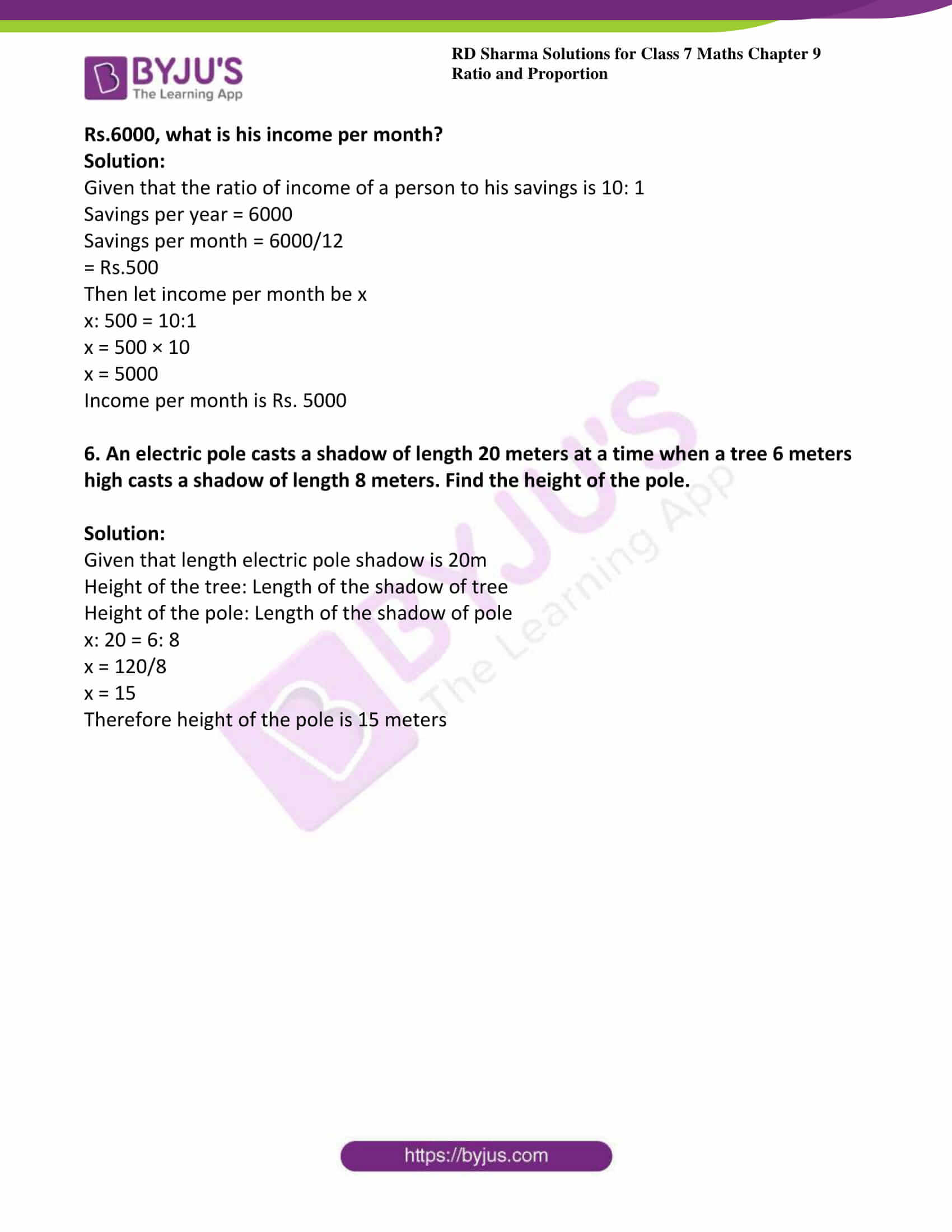# RD Sharma Solutions For Class 7 Maths Exercise 9.3 Chapter 9 Ratio And Proportion

In order to excel in the examinations, students can download the PDF of RD Sharma Solutions for Class 7 Maths Exercise 9.3 of Chapter 9 Ratio and Proportion. The PDF contains solutions for all questions present in the exercise solved by BYJU’S experts in Maths. By solving RD Sharma Solutions for Class 7, students will able to solve all problems individually without any dilemmas. This exercise explains the proportion, equality of two pairs. It also deals with continued proportion and mean proportional.

## Download the PDF of RD Sharma Solutions For Class 7 Maths Chapter 9 – Ratio and Proportion Exercise 9.3### Access answers to Maths RD Sharma Solutions For Class 7 Chapter 9 – Ratio and proportion Exercise 9.3

1. Find which of the following are in proportion?

(i) 33, 44, 66, 88

(ii) 46, 69, 69, 46

(iii) 72, 84, 186, 217

Solution:

(i) Given 33, 44, 66, 88

Product of extremes = 33 × 88 = 2904

Product of means = 44 × 66 = 2904

Therefore product of extremes = product of means

Hence given numbers are in proportion.

(ii) Given 46, 69, 69, 46

Product of extremes = 46 × 46 = 2116

Product of means = 69 × 69 = 4761

Therefore product of extremes is not equal to product of means

Hence given numbers are not in proportion.

(iii) Given 72, 84, 186, 217

Product of extremes = 72 × 217 = 15624

Product of means = 84 × 186 = 15624

Therefore product of extremes = product of means

Hence given numbers are in proportion.

2. Find x in the following proportions:

(i) 16: 18 = x: 96

(ii) x: 92 = 87: 116

Solution:

(i) Given 16: 18 = x: 96

In proportion we know that product of extremes = product of means

16/18 = x/96

On cross multiplying

x = (16 × 96)/ 18

x = 1536/18

Dividing both numerator and denominator by 6

x = 256/3

(ii) Given x: 92 = 87: 116

In proportion we know that product of extremes = product of means

x/ 92 = 87/116

On cross multiplying

x = (87 × 92)/ 116

x = 69

3. The ratio of income to the expenditure of a family is 7: 6. Find the savings if the income is Rs.1400.

Solution:

Given that income = 1400

Given the ratio of income and expenditure = 7: 6

7x = 1400

Therefore x = 200

Expenditure = 6x = 6 × 200 = Rs.1200

Savings = Income – Expenditure

= 1400 -1200

= Rs.200

4. The scale of a map is 1: 4000000. What is the actual distance between the two towns if they are 5cm apart on the map?

Solution:

Given that the scale of map = 1: 4000000

Let us assume the actual distance between towns is x cm

1: 4000000 =5: x

x = 5 × 4000000

x = 20000000 cm

We know that 1km = 1000 m

1m = 100 cm

Therefore

x = 200 km

5. The ratio of income of a person to his savings is 10: 1. If his savings for one year is Rs.6000, what is his income per month?

Solution:

Given that the ratio of income of a person to his savings is 10: 1

Savings per year = 6000

Savings per month = 6000/12

= Rs.500

Then let income per month be x

x: 500 = 10:1

x = 500 × 10

x = 5000

Income per month is Rs. 5000

6. An electric pole casts a shadow of length 20 meters at a time when a tree 6 meters high casts a shadow of length 8 meters. Find the height of the pole.

Solution:

Given that length electric pole shadow is 20m

Height of the tree: Length of the shadow of tree

Height of the pole: Length of the shadow of pole

x: 20 = 6: 8

x = 120/8

x = 15

Therefore height of the pole is 15 meters